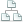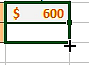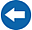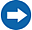Home >Tips >Excel 2016 from Scratch Tutorials >House costs calculation CSite map

# Excel 2016 from Scratch House Costs Calculation C

## An Excel tutorial by Peter KalmstromIn two earlier Tips articles in the Excel 2016 from Scratch series Peter Kalmström, CEO and Systems Designer of kalmstrom.com Business Solutions, has shown how to calculate house costs with the help of Excel formulas.

In the demo below, Peter continues with the house calculations, and he shows:
• How to calculate monthly and yearly interest costs with a formula that multiplies the values of two cells.
• How to create formulas that calculate sums in four different ways.
• How Excel tries to understand what you are doing and assist you with that.
• How to make cell content bold and larger.
Peter also repeats some useful Excel features shown in earlier demos in the Excel 2016 series:
• How to move cells and their content.
• How to insert a row.
• How to copy formatting from one cell to another.
•How to use the fill to enter the same kind of values and formatting in more cells.
• How to use Ctrl + z to undo changes.

Peter uses Excel 2016 for his demo, but the Excel basics are the same for earlier versions of Excel.

Do you want to try calculating sums? In next article there is a downloadable Excel file to work with, and Peter gives the solution in a demo.